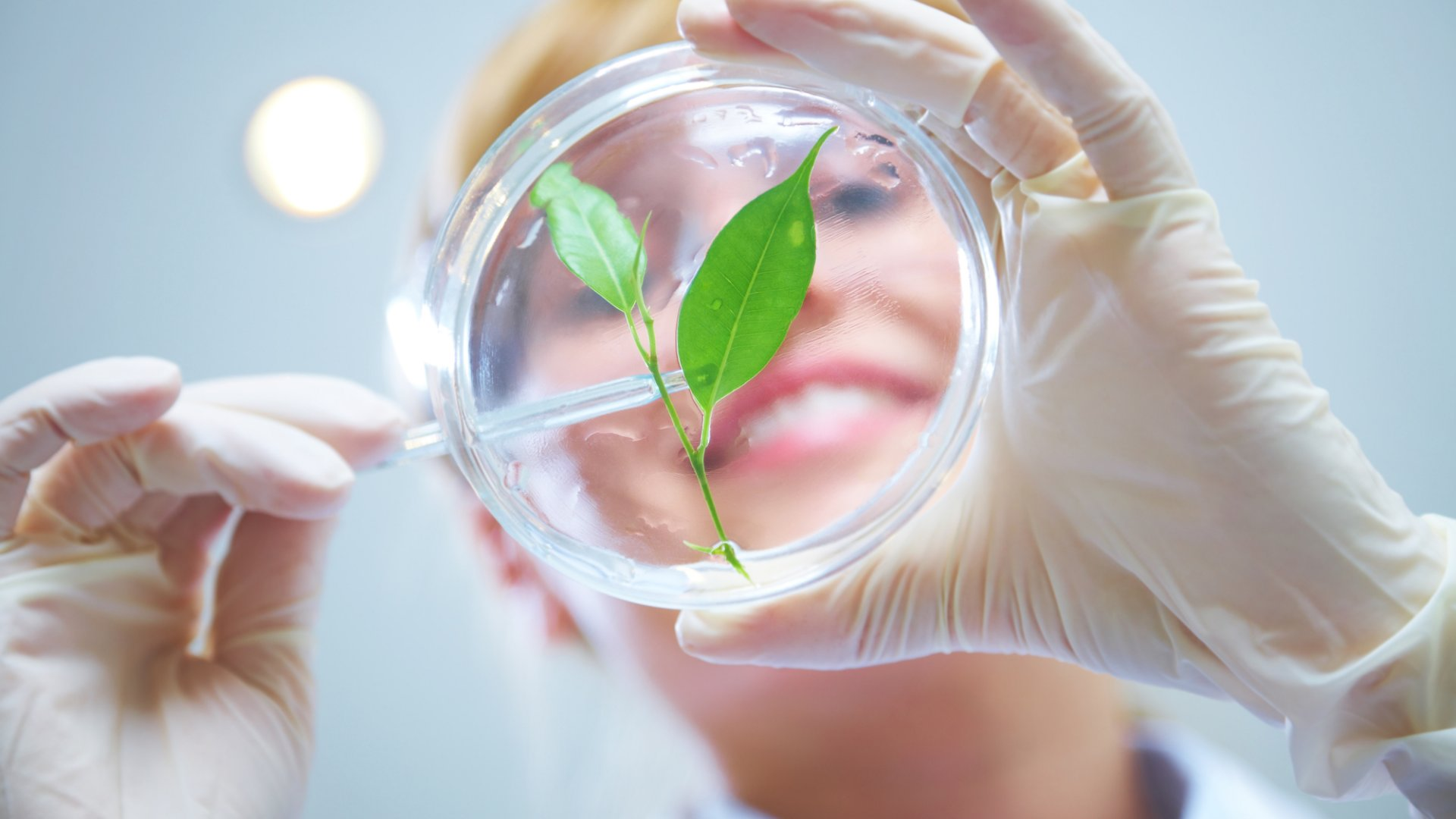Summer Sale! A-Levels now £399 & GCSEs £310! Sale ends in# Variables in Science

in Science January 1, 2020

Ok. You will be asked to draw a graph in IGCSE chemistry, physics, biology or science exams. In particular, you will be asked to draw a graph when you change one variable and measure what happens to the other variable.

But let’s back up a bit. I’ve gone and used a fancy science word – variables. We need to sort out this concept before we rush into drawing graphs.

A variable is just something you can measure and it is also something you can change. So, for example, the amount of sunlight a plant receives is a variable. We can change the amount it gets by taking it in and out of a dark cupboard. If we wanted to be really accurate we could also measure the amount of light it gets by using a meter.

Another variable would be the amount the plant grows.

These two variables are linked. The plant isn’t going to grow unless it receives sunlight. In fact the more sunlight we allow the plant to have then the more it should grow. The growth variable is dependent on the amount of sunlight variable.

For that reason the amount the plant grows is called the dependent variable. Guess what, the amount of sunlight, the bit we are controlling, is called the independent variable.

There is one other type of variable we need to look at. The so called control variables. These sound as if they have an important job, you know – controlling things. However, they don’t actually. Control variables are things that might affect what we are measuring, the dependent variable other than the thing we are changing (independent variable). So, in this case the amount of water the plant gets each day it is exposed to light or the temperature that is kept at during the experiment. These, and many other things, could affect the plant’s growth. We need to make sure that these variables are kept constant. In other words we need to make sure the plant gets the same amount of water each day. We also need to make sure it is kept at the same temperature.

## Why?

Well, if we didn’t then we would have no way of knowing if any changes in the plant’s growth were due to the amount of light it was getting or one of these other things.

So, we have to control these variables – we have to make sure that they are not changing.

It is important that you get your head round what these types of variables are. Not just for any IGCSE or GCSE courses but for A Level courses too. Some of you may be wanting to get a qualification to get access to a course at university, for example to get into a PGCE course for teacher training.

The main reason that I have written this post is because I have noticed on all the distance learning courses I have tutored on, whether they are online courses or by post, that many students find difficulty in this part of the work. Here at CloudLearn we want to ensure that our students are prepared for exam success.

In fact, some students may have to sit a practical exam or two, especially in courses such as A Level physics, chemistry and biology. In the practical exam you will be asked about variables.

Before we finish let’s have a quick look at possible variables in other courses.

In Online IGCSE Physics courses you might have to change the voltage across a circuit and measure the voltage in the circuit. In this case the voltage is the independent variable and the current is the dependent variable. Some of the control variables would be the temperature of the circuit and the resistance of the circuit.

In Online IGCSE Chemistry courses we might have an experiment where we want to measure the amount of bromine gas produced when we pass a current through a solution of lead bromide. In this case the independent variable is the amount of charge we put into the solution through the current and the dependent variable is the volume of gas given off. Control variables here would be the concentration of the lead bromide solution, the temperature and the volume of the solution.